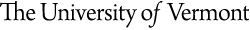University of Vermont

|Theoretical Condensed Matter Physics

Physics 301 Course Outline
Physics 301: Mathematical Physics
Course Outline

• Vector Analysis
• Curvilinear Coordinate Systems
• Calculus of Variations
• Orthogonal Functions
• Boundary Value Problems and Green's Functions
• Partial Differential Equations
• Integral Equations
• Complex Analysis
• Analytic Function Theory
• Conformal Mapping